# 4 matched filters and ambiguity functions for radar signals

18 de Jan de 2015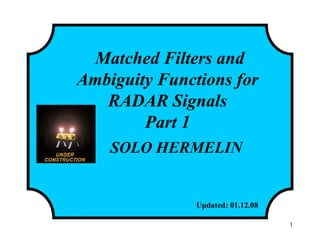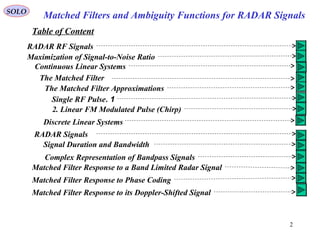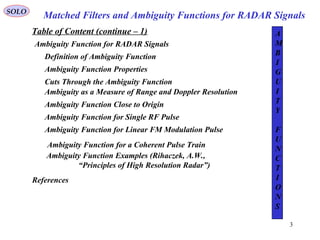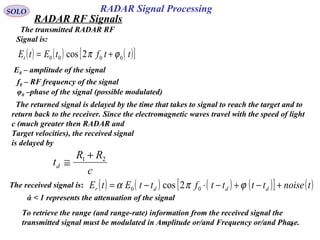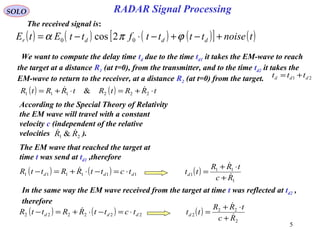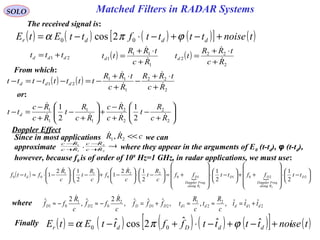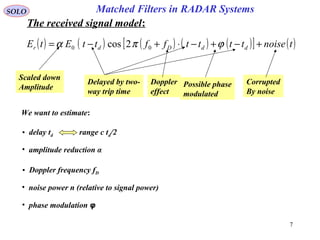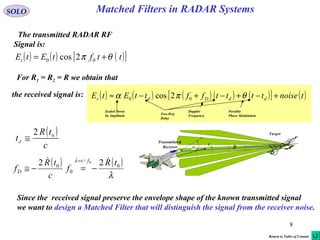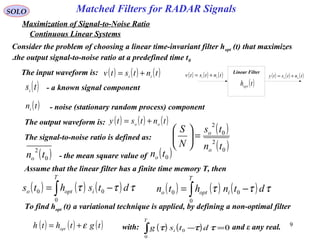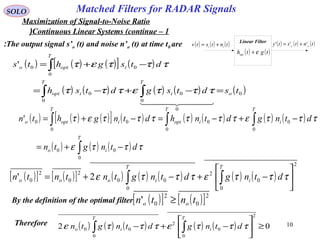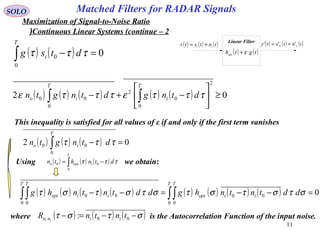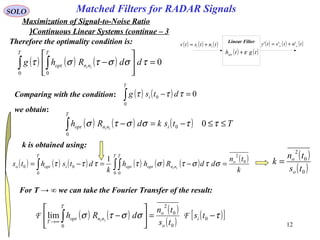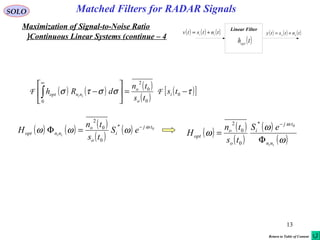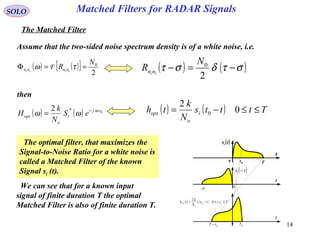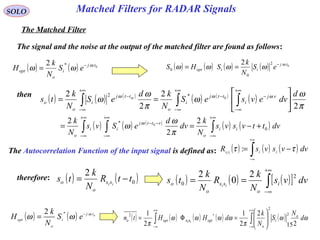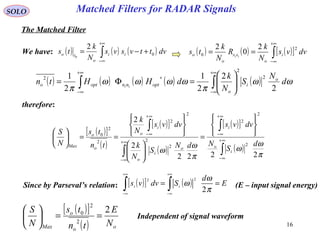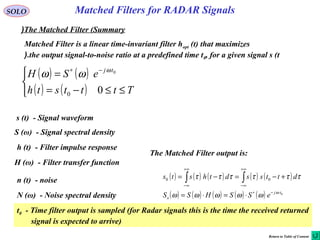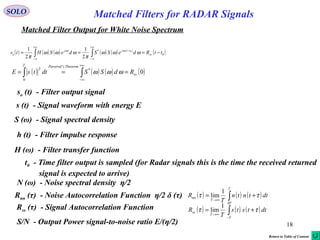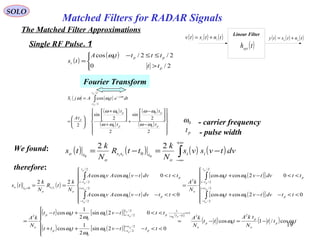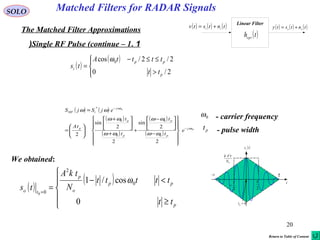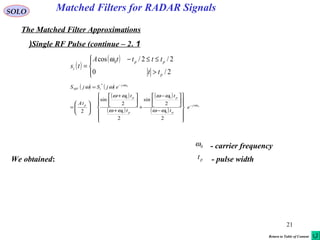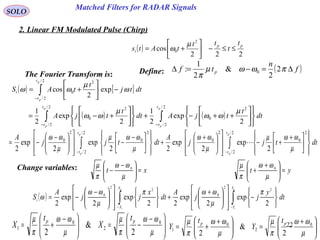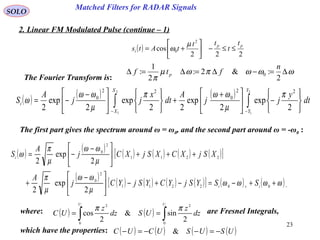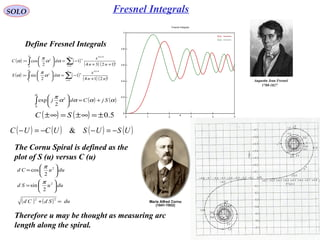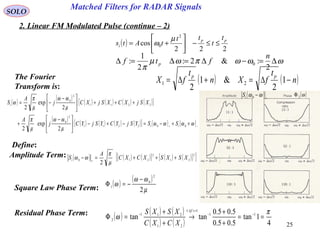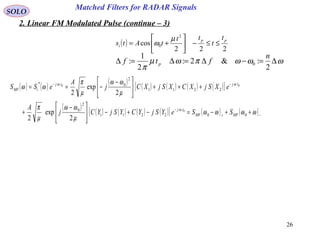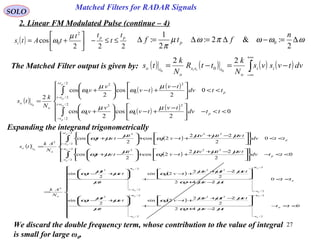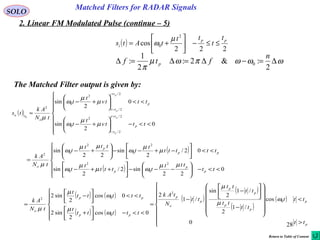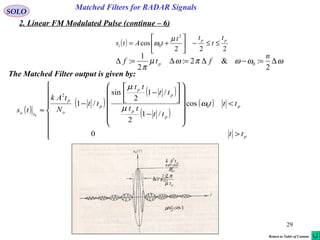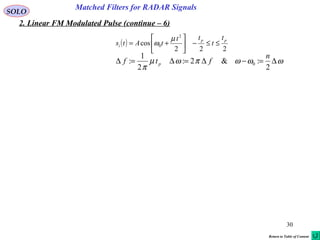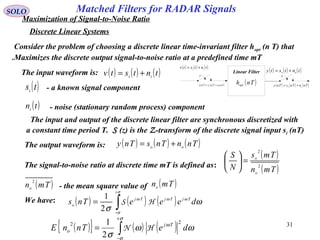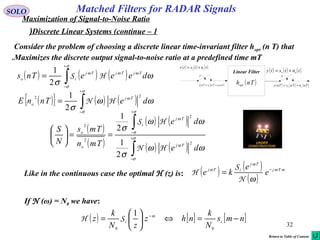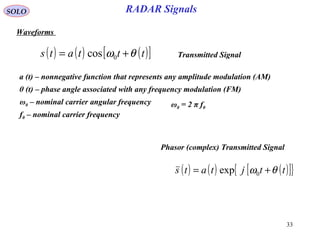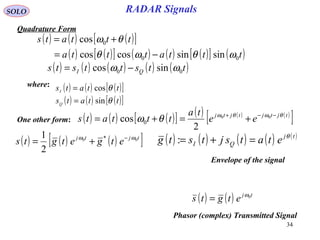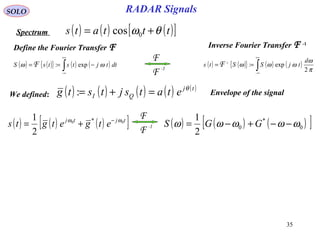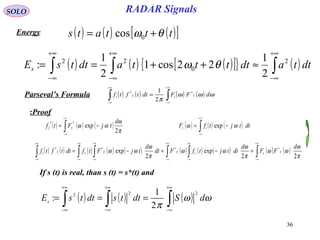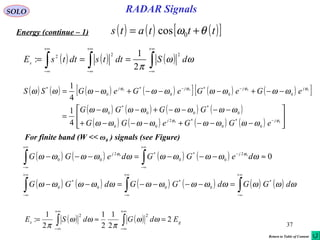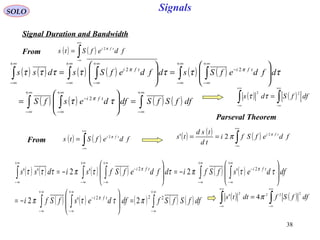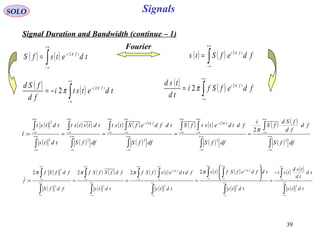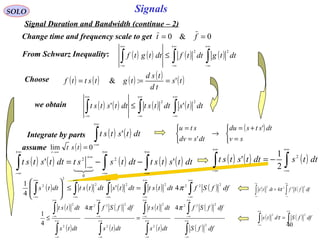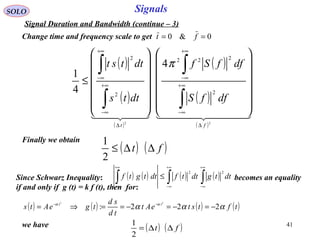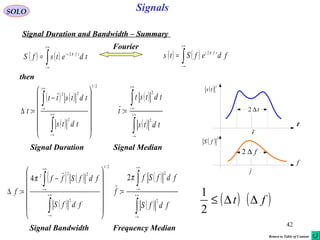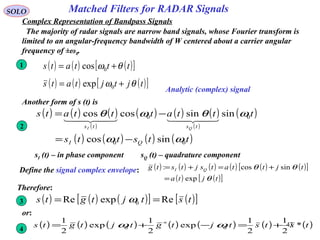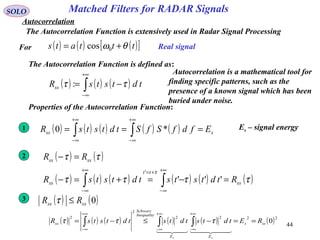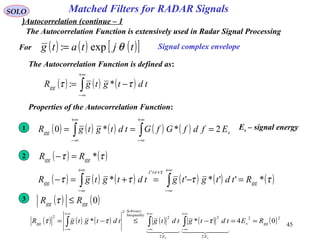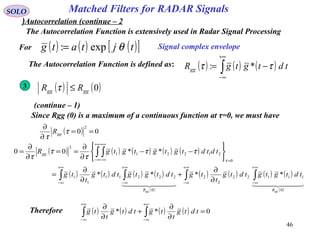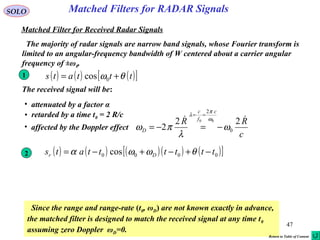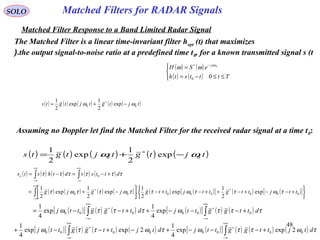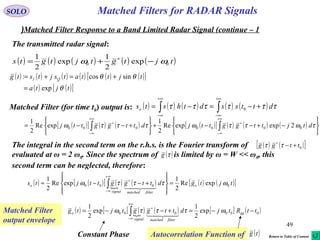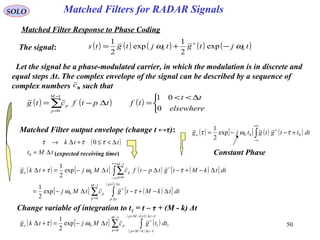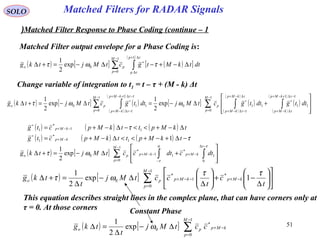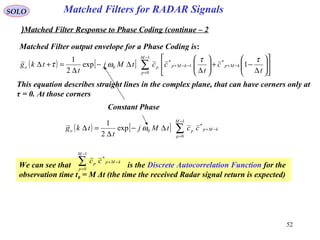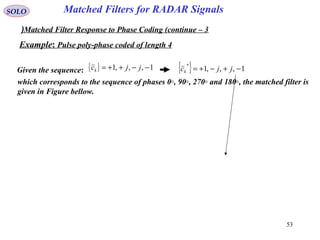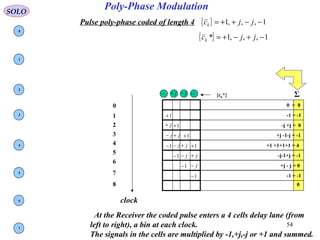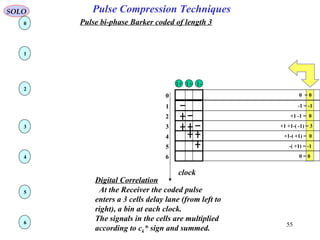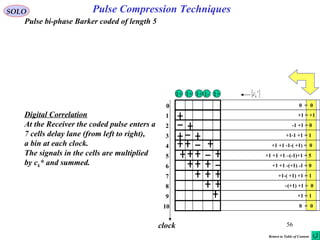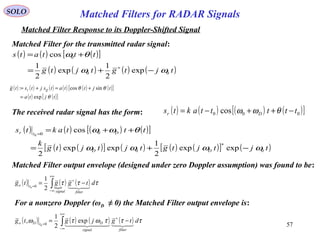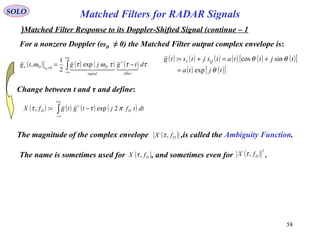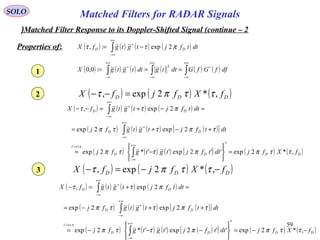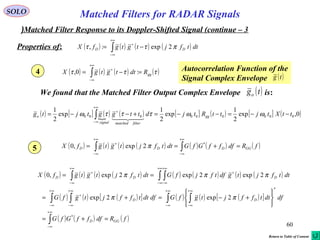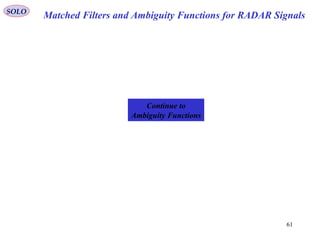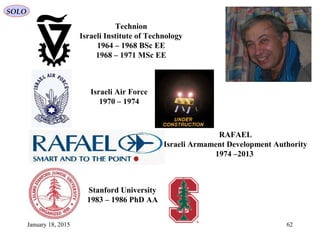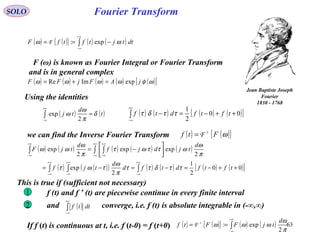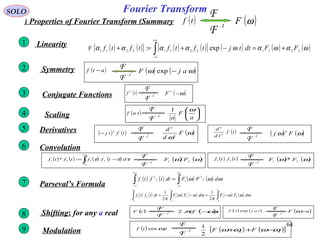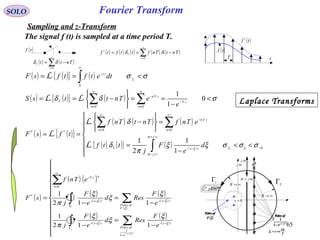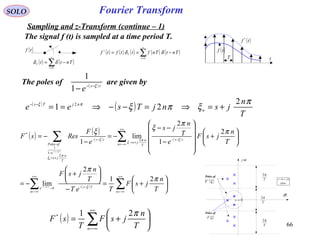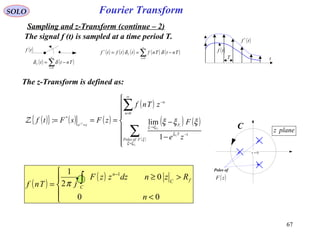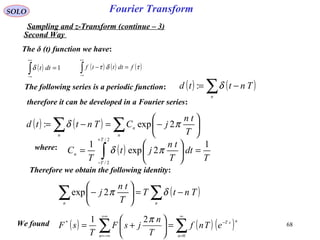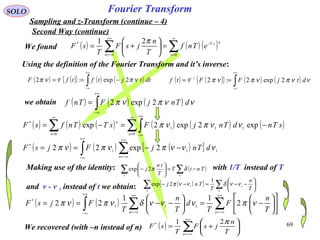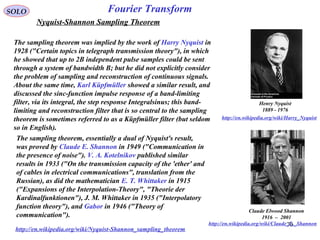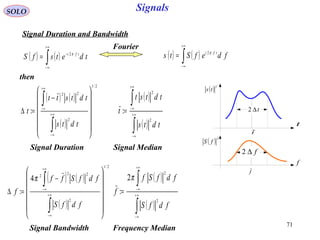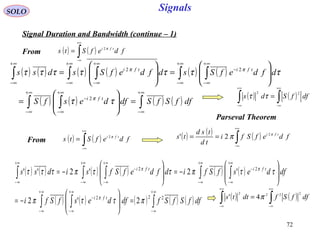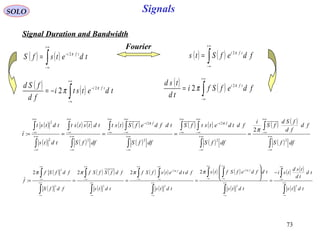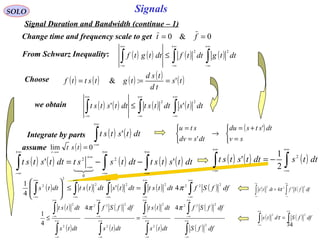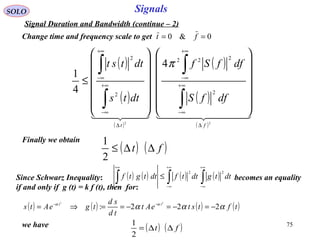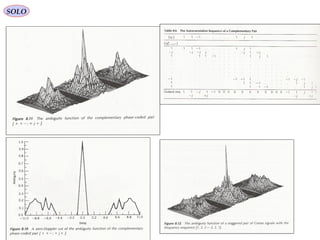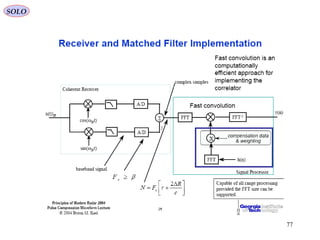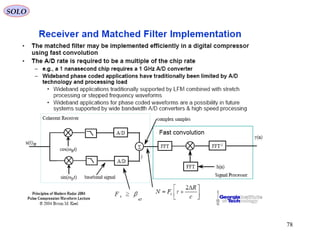1 de 78

### 4 matched filters and ambiguity functions for radar signals

• 1. 1 Matched Filters and Ambiguity Functions for RADAR Signals Part 1 SOLO HERMELIN Updated: 01.12.08http://www.solohermelin.com
• 2. 2 SOLO Matched Filters and Ambiguity Functions for RADAR Signals Table of Content RADAR RF Signals Maximization of Signal-to-Noise Ratio Continuous Linear Systems The Matched Filter The Matched Filter Approximations 1.Single RF Pulse 2. Linear FM Modulated Pulse (Chirp) Discrete Linear Systems RADAR Signals Signal Duration and Bandwidth Complex Representation of Bandpass Signals Matched Filter Response to a Band Limited Radar Signal Matched Filter Response to Phase Coding Matched Filter Response to its Doppler-Shifted Signal
• 3. 3 SOLO Matched Filters and Ambiguity Functions for RADAR Signals Table of Content (continue – 1) Ambiguity Function for RADAR Signals Definition of Ambiguity Function Ambiguity Function Properties Cuts Through the Ambiguity Function Ambiguity as a Measure of Range and Doppler Resolution Ambiguity Function Close to Origin Ambiguity Function for Single RF Pulse Ambiguity Function for Linear FM Modulation Pulse Ambiguity Function for a Coherent Pulse Train Ambiguity Function Examples (Rihaczek, A.W., “Principles of High Resolution Radar”) References A M B I G U I T Y F U N C T I O N S
• 4. 4 SOLO The transmitted RADAR RF Signal is: ( ) ( ) ( )[ ]ttftEtEt 0000 2cos ϕπ += E0 – amplitude of the signal f0 – RF frequency of the signal φ0 –phase of the signal (possible modulated) The returned signal is delayed by the time that takes to signal to reach the target and to return back to the receiver. Since the electromagnetic waves travel with the speed of light c (much greater then RADAR and Target velocities), the received signal is delayed by c RR td 21 + ≅ The received signal is: ( ) ( ) ( ) ( )[ ] ( )tnoisettttfttEtE dddr +−+−⋅−= ϕπα 00 2cos To retrieve the range (and range-rate) information from the received signal the transmitted signal must be modulated in Amplitude or/and Frequency or/and Phase. ά < 1 represents the attenuation of the signal RADAR Signal Processing RADAR RF Signals
• 5. 5 SOLO The received signal is: ( ) ( ) ( ) ( )[ ] ( )tnoisettttfttEtE dddr +−+−⋅−= ϕπα 00 2cos ( ) ( ) tRRtRtRRtR ⋅+=⋅+= 222111 &  We want to compute the delay time td due to the time td1 it takes the EM-wave to reach the target at a distance R1 (at t=0), from the transmitter, and to the time td2 it takes the EM-wave to return to the receiver, at a distance R2 (at t=0) from the target. 21 ddd ttt += According to the Special Theory of Relativity the EM wave will travel with a constant velocity c (independent of the relative velocities ).21 & RR  The EM wave that reached the target at time t was send at td1 ,therefore ( ) ( ) 111111 ddd tcttRRttR ⋅=−⋅+=−  ( ) 1 11 1 Rc tRR ttd   + ⋅+ = In the same way the EM wave received from the target at time t was reflected at td2 , therefore ( ) ( ) 222222 ddd tcttRRttR ⋅=−⋅+=−  ( ) 2 22 2 Rc tRR ttd   + ⋅+ = RADAR Signal Processing
• 6. 6 SOLO The received signal is: ( ) ( ) ( ) ( )[ ] ( )tnoisettttfttEtE dddr +−+−⋅−= ϕπα 00 2cos 21 ddd ttt += ( ) 1 11 1 Rc tRR ttd   + ⋅+ = ( ) 2 22 2 Rc tRR ttd   + ⋅+ = ( ) ( ) 2 22 1 11 21 Rc tRR Rc tRR tttttttt ddd     + ⋅+ − + ⋅+ −=−−=−       + − + − +      + − + − =− 2 2 2 2 1 1 1 1 2 1 2 1 Rc R t Rc Rc Rc R t Rc Rc tt d     From which: or: Since in most applications we can approximate where they appear in the arguments of E0 (t-td), φ (t-td), however, because f0 is of order of 109 Hz=1 GHz, in radar applications, we must use: cRR <<21,  1, 2 2 1 1 ≈ + − + − Rc Rc Rc Rc     ( )         −⋅           ++      −⋅           +=      −⋅      −+      −⋅      −⋅≈− 2 . 201 . 10 22 0 11 00 2 1 2 1 2 12 1 2 12 1 21 D Ralong FreqDoppler DD Ralong FreqDoppler Dd ttffttff c R t c R f c R t c R fttf  ( ) ( ) ( ) ( ) ( )[ ] ( )tnoisettttffttEtE ddDdr +−+−⋅+−= ˆˆˆ2cosˆ 00 ϕπα where 21 2 2 1 121 2 02 1 01 ˆˆˆ,,,ˆˆˆ, 2ˆ, 2ˆ dddddDDDDD ttt c R t c R tfff c R ff c R ff +=≈≈+=−≈−≈  Finally Matched Filters in RADAR Systems Doppler Effect
• 7. 7 SOLO The received signal model: ( ) ( ) ( ) ( ) ( )[ ] ( )tnoisettttffttEtE ddDdr +−+−⋅+−= ϕπα 00 2cos Matched Filters in RADAR Systems Delayed by two- way trip time Scaled down Amplitude Possible phase modulated Corrupted By noise Doppler effect We want to estimate: • delay td range c td/2 • amplitude reduction α • Doppler frequency fD • noise power n (relative to signal power) • phase modulation φ
• 8. 8 Matched Filters in RADAR SystemsSOLO α MV R EV Target Transmitter& Receiver The transmitted RADAR RF Signal is: ( ) ( ) ( )[ ]ttftEtEt θπ += 00 2cos ( ) c tR td 02 ≅ Since the received signal preserve the envelope shape of the known transmitted signal we want to design a Matched Filter that will distinguish the signal from the receiver noise. ( ) ( ) λ λ 0 / 0 0 22 0 tR f c tR f fc D  −=−≅ = the received signal is: ( ) ( ) ( ) ( ) ( )[ ] ( )tnoisettttffttEtE ddDdr +−+−+−≈ θπα 00 2cos Scaled Down In Amplitude Two-Way Delay Possible Phase Modulation Doppler Frequency For R1 = R2 = R we obtain that Return to Table of Content
• 9. 9 Matched Filters for RADAR Signals ( ) ( ) ( )tntstv ii += Linear Filter ( )thopt ( ) ( ) ( )tntsty oo += SOLO Maximization of Signal-to-Noise Ratio Consider the problem of choosing a linear time-invariant filter hopt (t) that maximizes the output signal-to-noise ratio at a predefined time t0. The input waveform is: ( ) ( ) ( )tntstv ii += ( )tsi - a known signal component ( )tni - noise (stationary random process) component The output waveform is: ( ) ( ) ( )tntsty oo += Assume that the linear filter has a finite time memory T, then ( ) ( ) ( )∫ −= T iopto dtshts 0 00 τττ ( ) ( ) ( )∫ −= T iopto dtnhtn 0 00 τττ The signal-to-noise ratio is defined as: ( ) ( )0 2 0 2 tn ts N S o o =      To find hopt (t) a variational technique is applied, by defining a non-optimal filter ( ) ( ) ( )tgthth opt ε+= ( ) ( ) 0 0 0 =−∫ T i dtsg τττwith: and ε any real. ( )0 2 tno - the mean square value of ( )0tno Continuous Linear Systems
• 10. 10 Matched Filters for RADAR Signals ( ) ( ) ( )tntstv ii += Linear Filter ( ) ( )tgthopt ε+ ( ) ( ) ( )tntsty oo ''' += SOLO Maximization of Signal-to-Noise Ratio The output signal s’o (t) and noise n’o (t) at time t0 are: ( ) ( ) ( )[ ] ( ) ( ) ( ) ( ) ( ) ( )0 0 0 0 0 0 0 00' tsdtsgdtsh dtsghts o T i T iopt T iopto =−+−= −+= ∫∫ ∫    τττετττ τττετ ( ) ( ) ( )[ ] ( ) ( ) ( ) ( ) ( ) ( ) ( ) ( )∫ ∫∫∫ −+= −+−=−+= T io T i T iopt T iopto dtngtn dtngdtnhdtnghtn 0 00 0 0 0 0 0 00' τττε τττεττττττετ ( )[ ] ( )[ ] ( ) ( ) ( ) ( ) ( ) 2 0 0 2 0 00 2 0 2 0 2'       −+−+= ∫∫ T i T iooo dtngdtngtntntn τττετττε By the definition of the optimal filter ( )[ ] ( )[ ]2 0 2 0' tntn oo ≥ Therefore ( ) ( ) ( ) ( ) ( ) 02 2 0 0 2 0 00 ≥      −+− ∫∫ T i T io dtngdtngtn τττετττε Continuous Linear Systems (continue – 1(
• 11. 11 Matched Filters for RADAR Signals ( ) ( ) ( )tntstv ii += Linear Filter ( ) ( )tgthopt ε+ ( ) ( ) ( )tntsty oo ''' += SOLO Maximization of Signal-to-Noise Ratio This inequality is satisfied for all values of ε if and only if the first term vanishes ( ) ( ) ( ) ( ) ( ) 02 2 0 0 2 0 00 ≥      −+− ∫∫ T i T io dtngdtngtn τττετττε ( ) ( ) 0 0 0 =−∫ T i dtsg τττ ( ) ( ) ( ) 02 0 00 =−∫ T io dtngtn τττ Using we obtain:( ) ( ) ( )∫ −= T iopto dtnhtn 0 00 τττ ( ) ( ) ( ) ( ) ( ) ( ) ( ) ( ) 0 0 0 00 0 0 00 =−−=−− ∫∫∫∫ T T iiopt T T iiopt ddtntnhgddtntnhg στστστστστστ where is the Autocorrelation Function of the input noise.( ) ( ) ( )στστ −−=− 00: tntnR iinn ii Continuous Linear Systems (continue – 2(
• 12. 12 Matched Filters for RADAR Signals ( ) ( ) ( )tntstv ii += Linear Filter ( ) ( )tgthopt ε+ ( ) ( ) ( )tntsty oo ''' += SOLO Maximization of Signal-to-Noise Ratio Therefore the optimality condition is: ( ) ( ) ( ) 0 0 0 =      −∫ ∫ τσστστ ddRhg T T nnopt ii ( ) ( ) 0 0 0 =−∫ T i dtsg τττComparing with the condition: we obtain: ( ) ( ) ( ) TtskdRh i T nnopt ii ≤≤−=−∫ ττσστσ 00 0 k is obtained using: ( ) ( ) ( ) ( ) ( ) ( ) ( ) k tn ddRhh k dtshts o T T nnoptopt T iopto ii 0 2 0 00 00 1 =−=−= ∫∫∫ στστσττττ ( ) ( )0 0 2 ts tn k o o = For T → ∞ we can take the Fourier Transfer of the result: ( ) ( ) ( ) ( ) ( )[ ]τσστσ −=      −∫∞→ 0 0 0 2 0 lim ts ts tn dRh i o o T nnopt T ii FF Continuous Linear Systems (continue – 3(
• 13. 13 Matched Filters for RADAR SignalsSOLO Maximization of Signal-to-Noise Ratio ( ) ( ) ( ) ( ) ( )[ ]τσστσ −=      −∫ ∞ 0 0 0 2 0 ts ts tn dRh i o o nnopt ii FF ( ) ( ) ( )tntstv ii += Linear Filter ( )thopt ( ) ( ) ( )tntsty oo += ( ) ( ) ( ) ( ) ( ) 0* 0 0 2 tj i o o nnopt eS ts tn H ii ω ωωω − =Φ ( ) ( ) ( ) ( ) ( )ω ω ω ω iinn tj i o o opt eS ts tn H Φ = − 0* 0 0 2 Continuous Linear Systems (continue – 4( Return to Table of Content
• 14. 14 Matched Filters for RADAR Signals ( )tsi t T0 mt SOLO The Matched Filter Assume that the two-sided noise spectrum density is of a white noise, i.e. ( ) ( )στδστ −=− 2 0N R iinn ( ) ( )[ ] 2 0N R iiii nnnn ==Φ τω F then ( ) ( ) 0*2 tj i o opt eS N k H ω ωω − = ( ) ( ) Tttts N k th i o opt ≤≤−= 0 2 0 ( )tsi t t ( )tsi − T0 0 T− mt ( )tsi t t t ( )tsi − ( ) ( ) Ttttts N k th mmiopt ≤≤−= ,0 2 0 T0 0 T− 0 mtm tT − mt The optimal filter, that maximizes the Signal-to-Noise Ratio for a white noise is called a Matched Filter of the known Signal si (t). We can see that for a known input signal of finite duration T the optimal Matched Filter is also of finite duration T.
• 15. 15 Matched Filters for RADAR Signals ( ) ( ) ( ) ( ) 0 2 0 0 2 tj iiopt eS N k SHS ω ωωωω − == SOLO The Matched Filter The signal and the noise at the output of the matched filter are found as follows: then ( ) ( ) ( ) ( ) ( ) ( )∫ ∫∫ +∞ ∞− +∞ ∞− −− +∞ ∞− −       == π ω ω π ω ω ωωω 2 2 2 2 0*2 d dvevseS N kd eS N k ts vj i ttj i o ttj i o o m ( ) ( ) 0*2 tj i o opt eS N k H ω ωω − = ( ) ( ) ( ) ( ) ( )∫∫ ∫ +∞ ∞− +∞ ∞− +∞ ∞− −− +−== dvttvsvs N k dv d eSvs N k ii o vttj ii o 0 * 2 2 2 0 π ω ω ω The Autocorrelation Function of the input signal is defined as: ( ) ( ) ( )∫ +∞ ∞− −= dvvsvsR iiss ii ττ : therefore: ( ) ( )0 2 ttR N k ts iiss o o −= ( ) ( ) ( ) ( ) ( )∫∫ +∞ ∞− +∞ ∞− ∗       =Φ= ωω π ωωωω π d N S N k dHHtn o i o optnnopto ii 2 2 2 1 2 1 2 2 2 ( ) ( ) mtj i o opt eS N k H ω ωω − = *2 ( ) ( ) ( )[ ]∫ +∞ ∞− == dvvs N k R N k ts i o ss o o ii 2 0 2 0 2
• 16. 16 Matched Filters for RADAR SignalsSOLO The Matched Filter therefore: ( ) ( ) ( ) ( ) ( )∫∫ +∞ ∞− +∞ ∞− ∗       =Φ= ωω π ωωωω π d N S N k dHHtn o i o optnnopto ii 2 2 2 1 2 1 2 2 2 ( ) ( ) ( )[ ]∫ +∞ ∞− == dvvs N k R N k ts i o ss o o ii 2 0 2 0 2 ( )[ ] ( ) ( )[ ] ( ) ( )[ ] ( )∫ ∫ ∫ ∫ ∞+ ∞− ∞+ ∞− ∞+ ∞− ∞+ ∞−       =             ==      π ω ω π ω ω 2222 2 2 2 2 2 2 2 2 2 2 2 0 d S N dvvs dN S N k dvvs N k tn ts N S i o i o i o i o o o Max Since by Parseval’s relation: (E – input signal energy)( )[ ] ( ) E d Sdvvs ii == ∫∫ +∞ ∞− +∞ ∞− π ω ω 2 22 ( )[ ] ( ) oo o Max N E tn ts N S 2 2 2 0 ==      We have: ( ) ( ) ( )∫ +∞ ∞− +−= dvttvsvs N k ts ii o to 0 2 0 Independent of signal waveform
• 17. 17 Matched Filters for RADAR Signals ( ) ( ) ( ) ( )   ≤≤−= = −∗ Ttttsth eSH tj 00 0ω ωω SOLO The Matched Filter (Summary( s (t) - Signal waveform S (ω) - Signal spectral density h (t) - Filter impulse response H (ω) - Filter transfer function t0 - Time filter output is sampled (for Radar signals this is the time the received returned signal is expected to arrive) n (t) - noise N (ω) - Noise spectral density Matched Filter is a linear time-invariant filter hopt (t) that maximizes the output signal-to-noise ratio at a predefined time t0, for a given signal s (t(. The Matched Filter output is: ( ) ( ) ( ) ( ) ( ) ( ) ( ) ( ) ( ) ( ) 0 00 tj o eSSHSS dttssdthsts ω ωωωωω ττττττ −∗ +∞ ∞− +∞ ∞− ⋅=⋅= +−=−= ∫∫ Return to Table of Content
• 18. 18 Matched Filters for RADAR SignalsSOLO Matched Filter Output for White Noise Spectrum s (t) - Signal waveform with energy E S (ω) - Signal spectral density h (t) - Filter impulse response H (ω) - Filter transfer function ( ) ( ) ( ) ( ) ( ) ( ) ( )0 * 0 2 1 2 1 ttRdeSSdeSHts ss ttjtj o −=== ∫∫ +∞ ∞− − +∞ ∞− ωωω π ωωω π ωω ( ) ( ) ( ) ( )0* ' 0 2 ss TheoremsParsevalT RdSSdttsE === ∫∫ +∞ ∞− ωωω so (t) - Filter output signal N (ω) - Noise spectral density η/2 Rnn (τ) - Noise Autocorrelation Function η/2 δ (τ) ( ) ( ) ( )∫− ∞→ += T T T nn dttntn T R ττ 1 lim Rss (τ) - Signal Autocorrelation Function ( ) ( ) ( )∫− ∞→ += T T T ss dttsts T R ττ 1 lim S/N - Output Power signal-to-noise ratio E/(η/2) t0 - Time filter output is sampled (for Radar signals this is the time the received returned signal is expected to arrive) Return to Table of Content
• 19. 19 Matched Filters for RADAR Signals SOLO The Matched Filter Approximations 1.Single RF Pulse ( ) ( )     > ≤≤− = 2/0 2/2/cos 0 p pp i tt ttttA ts ω pt - pulse width ( ) ( ) ( ) ( ) ( ) ( )               −       − + +       +       = = ∫− − 2 2 sin 2 2 sin 2 cos 0 0 0 0 2/ 2/ 0 p p p p p t t tj i t t t t tA dtetAjS p p ωω ωω ωω ωω ωω ω Fourier Transform 0ω - carrier frequency We found: ( ) ( ) ( ) ( )∫ +∞ ∞− −=−= dvtvsvs N k ttR N k ts ii o tss o to ii 22 00 0 therefore: ( ) ( ) ( ) ( ) ( )[ ] ( )[ ] ( ) ( )[ ] ( ) ( )[ ] ( ) ( ) ( ) ttt N tkA ttt N kA tttvttt tttvttt N kA ttdvtvt ttdvtvt N kA ttdvtvAvA ttdvtvAvA N k tR N k ts p o p p o tt p tt tp p t ttp o p tt t p t tt o tt t p p t tt o ss o to p p p p p p p p p p p p p ii 0 2 0 2 1 1 2/ 2/0 0 0 2/ 2/0 0 02 2/ 2/ 00 2/ 2/ 00 2 2/ 2/ 00 2/ 2/ 00 0 cos/1cos 02sin 2 1 cos 02sin 2 1 cos 02coscos 02coscos 0coscos 0coscos 22 0 2 0 ωω ω ω ω ω ω ω ωω ωω ωω ωω ω −=−≈        <<−−++ <<−+− =         <<−−+ <<−+ =         <<−− <<− == << − + − − + − − + − − = ∫ ∫ ∫ ∫ ( ) ( ) ( )tntstv ii += Linear Filter ( )thopt ( ) ( ) ( )tntsty oo +=
• 20. 20 Matched Filters for RADAR SignalsSOLO The Matched Filter Approximations 1.Single RF Pulse (continue – 1( ( ) ( )     > ≤≤− = 2/0 2/2/cos 0 p pp i tt ttttA ts ω pt - pulse width ( ) ( ) ( ) ( ) ( ) ( ) 0 0 2 2 sin 2 2 sin 2 0 0 0 0 * tj p p p p p tj iMF e t t t t tA ejSjS ω ω ωω ωω ωω ωω ωω − −               −       − + +       +       = = 0ω - carrier frequency We obtained: ( ) ( )      ≥ <− == p pp o p to tt ttttt N tkA ts 0 cos/1 0 2 00 ω ( ) ( ) ( )tntstv ii += Linear Filter ( )thopt ( ) ( ) ( )tntsty oo += t 2 τ 2 τ − ( )tso 0 2 N Ak τ ττ− 0=mt Return to Table of Content
• 21. 21 Matched Filters for RADAR SignalsSOLO The Matched Filter Approximations 1.Single RF Pulse (continue – 2( ( ) ( )     > ≤≤− = 2/0 2/2/cos 0 p pp i tt ttttA ts ω pt - pulse width ( ) ( ) ( ) ( ) ( ) ( ) 0 0 2 2 sin 2 2 sin 2 0 0 0 0 * tj p p p p p tj iMF e t t t t tA ejSjS ω ω ωω ωω ωω ωω ωω − −               −       − + +       +       = = 0ω - carrier frequency We obtained: Return to Table of Content
• 22. 22 SOLO 2. Linear FM Modulated Pulse (Chirp) ( ) 222 cos 2 0 pp i t t tt tAts ≤≤−      += µ ω The Fourier Transform is: ( ) [ ] ( ) ( )∫∫ ∫ −− −             ++−+             +−= −      += 2/ 2/ 2 0 2/ 2/ 2 0 2/ 2/ 2 0 2 exp 2 1 2 exp 2 1 exp 2 cos p p p p p p t t t t t t i dt t tjAdt t tjA dttj t tAS µ ωω µ ωω ω µ ωω ∫∫ −−               + +−               + +               − −               − −= 2/ 2/ 2 0 2 0 2/ 2/ 2 0 2 0 2 exp 2 exp 22 exp 2 exp 2 p p p p t t t t dttjj A dttjj A µ ωωµ µ ωω µ ωωµ µ ωω  Change variables: xt =      − − µ ωω π µ 0 yt =      + + µ ωω π µ 0 ( ) ∫∫ −−       −               + +                     − −= 2 1 2 1 2 exp 2 exp 22 exp 2 exp 2 2 2 0 2 2 0 Y Y X X i dt y jj A dt x jj A S π µ ωωπ µ ωω ω       − −=      − += µ ωω π µ µ ωω π µ 0 2 0 1 2 & 2 pp t X t X       + −=      + += µ ωω π µ µ ωω π µ 0 2 0 1 2 & 2 pp t Y t Y Define: ( )f n tf p ∆=−=∆ πωωµ π 2 2 & 2 1 : 0 Matched Filters for RADAR Signals
• 23. 23 SOLO 2. Linear FM Modulated Pulse (continue – 1) The Fourier Transform is: ( ) ( ) ( ) ∫∫ −−       −      + +             − −= 2 1 2 1 2 exp 2 exp 22 exp 2 exp 2 22 0 22 0 Y Y X X i dt y jj A dt x jj A S π µ ωωπ µ ωω ω The first part gives the spectrum around ω = ω0, and the second part around ω = -ω0 : where: are Fresnel Integrals, which have the properties: ( ) ( ) ∫∫ == UU dz z USdz z UC 0 2 0 2 2 sin& 2 cos ππ ( ) ( ) ( ) ( )USUSUCUC −=−−=− & ( ) ( ) ( ) ( ) ( ) ( )[ ] ( ) ( ) ( ) ( ) ( )[ ] ( ) ( )−+ ++−=−+−      − + +++      − −= ωωωω µ ωω µ π µ ωω µ π ω 002211 2 0 2211 2 0 2 exp 2 2 exp 2 ii i SSYSjYCYSjYCj A XSjXCXSjXCj A S Matched Filters for RADAR Signals ( ) 222 cos 2 0 pp i t t tt tAts ≤≤−      += µ ω ωωωπωµ π ∆=−∆=∆=∆ 2 :&2: 2 1 : 0 n ftf p
• 24. 24 SOLO Fresnel Integrals Augustin Jean Fresnel 1788-1827 Define Fresnel Integrals ( ) ( ) ( ) ( ) ( ) ( ) ( ) ( )∫ ∑ ∑∫ ∞ = + ∞ = + + −=      = ++ −=      = α α αα π α αα π α 0 0 14 2 0 34 0 2 !214 1 2 sin: !1234 1 2 cos: n n n n n n nn x dS nn x dC ( ) ( )αααα πα SjCdj +=      ∫0 2 2 exp ( ) ( ) 5.0±=∞±=∞± SC ( ) ( ) ( ) ( )USUSUCUC −=−−=− & The Cornu Spiral is defined as the plot of S (u) versus C (u) duuSd duuCd       =       = 2 2 2 sin 2 cos π π ( ) ( ) duSdCd =+ 22 Therefore u may be thought as measuring arc length along the spiral.
• 25. 25 SOLO 2. Linear FM Modulated Pulse (continue – 2) The Fourier Transform is: Define: ( ) ( ) ( )[ ] ( ) ( )[ ]{ }2 21 2 210 2 XSXSXCXC A Si +++=− + µ π ωωAmplitude Term: Square Law Phase Term: ( ) ( ) µ ωω ω 2 2 0 1 − −=Φ Residual Phase Term: ( ) ( ) ( ) ( ) ( ) 4 1tan 5.05.0 5.05.0 tantan 11 1 21 211 2 π ω τ == + + → + + =Φ −− >>∆ − f XCXC XSXS ( ) ( )n t fXn t fX pp −∆=+∆= 1 2 &1 2 21 ( )ω2Φ( ) + − ωω0iS ( ) ( ) ( ) ( ) ( ) ( )[ ] ( ) ( ) ( ) ( ) ( )[ ] ( ) ( )−+ ++−=−+−      − + +++      − −= ωωωω µ ωω µ π µ ωω µ π ω 002211 2 0 2211 2 0 2 exp 2 2 exp 2 ii i SSYSjYCYSjYCj A XSjXCXSjXCj A S Matched Filters for RADAR Signals ( ) 222 cos 2 0 pp i t t tt tAts ≤≤−      += µ ω ωωωπωµ π ∆=−∆=∆=∆ 2 :&2: 2 1 : 0 n ftf p
• 26. 26 SOLO 2. Linear FM Modulated Pulse (continue – 3) Matched Filters for RADAR Signals ( ) 222 cos 2 0 pp i t t tt tAts ≤≤−      += µ ω ωωωπωµ π ∆=−∆=∆=∆ 2 :&2: 2 1 : 0 n ftf p ( ) ( ) ( ) ( ) ( ) ( ) ( )[ ] ( ) ( ) ( ) ( ) ( )[ ] ( ) ( )−+ − −− ++−=−+−      − + +++      − −== ωωωω µ ωω µ π µ ωω µ π ωω ω ωω 002211 2 0 2211 2 0* 0 00 2 exp 2 2 exp 2 MFMF tj tjtj iMF SSeYSjYCYSjYCj A eXSjXCXSjXCj A eSS
• 27. 27 SOLO 2. Linear FM Modulated Pulse (continue – 4) Matched Filters for RADAR Signals ( ) 222 cos 2 0 pp i t t tt tAts ≤≤−      += µ ω ωωωπωµ π ∆=−∆=∆=∆ 2 :&2: 2 1 : 0 n ftf p The Matched Filter output is given by: ( ) ( ) ( ) ( )∫ +∞ ∞− −=−= dvtvsvs N k ttR N k ts ii o tss o to ii 22 00 0 ( ) ( ) ( ) ( ) ( )         <<−      − +−      + <<      − +−      + = ∫ ∫ + − + − 2/ 2/ 2 0 2 0 2/ 2/ 2 0 2 0 0 2 cos 2 cos 0 2 cos 2 cos 2 0 p p p p tt t p t tt p o to ttdv tv tv v v ttdv tv tv v v N k ts µ ω µ ω µ ω µ ω We discard the double frequency term, whose contribution to the value of integral is small for large ω0, ( ) ( ) ( )         <<−             −+ +−+      −+ <<             −+ +−+      −+ = ∫ ∫ + − + − 2/ 2/ 22 0 2 0 2/ 2/ 22 0 2 0 2 0 2 22 2cos 2 cos 0 2 22 2cos 2 cos 0 p p p p tt t p t tt p o to ttdv tvtv tv t tvt ttdv tvtv tv t tvt N Ak ts µµµ ω µ µω µµµ ω µ µω ( ) ( )                <<− −+       −+ +− +       +− << −+       −+ +− +       +− = + − + − + − + − 0 242 2 22 2sin 2 sin 0 242 2 22 2sin 2 sin 2/ 2/ 0 22 0 2/ 2/ 2 0 2/ 2/ 0 22 0 2/ 2/ 2 0 2 tt tv tvtv tv t tv t t tt tv tvtv tv t tv t t N Ak p tt t tt t p t tt t tt o p p p p p p p p µµω µµµω µ µµω µµω µµµω µ µµω Expanding the integrand trigonometrically
• 28. 28 SOLO 2. Linear FM Modulated Pulse (continue – 5) Matched Filters for RADAR Signals Return to Table of Content ( ) 222 cos 2 0 pp i t t tt tAts ≤≤−      += µ ω ωωωπωµ π ∆=−∆=∆=∆ 2 :&2: 2 1 : 0 n ftf p The Matched Filter output is given by: ( )          <<−      +− <<      +− ≈ + − + − 0 2 sin 0 2 sin 2/ 2/ 2 0 2/ 2/ 2 0 2 0 tttv t t tttv t t tN Ak ts p tt t p t tt o to p p p p µ µ ω µ µ ω µ ( ) ( )       <<−      −−−      ++− <<      −+−−      +− = 0 22 sin2/ 2 sin 02/ 2 sin 22 sin 2 0 2 0 2 0 2 02 tt ttt tttt t t ttttt t t ttt t tN Ak p p p pp p o µµ ωµ µ ω µ µ ω µµ ω µ ( ) ( ) ( ) ( ) ( ) ( ) ( ) ( )          > <               −       − − =        <<−      + <<      − = p p p p p p p o p pp pp o tt ttt tt tt tt tt tt N tAk ttttt t ttttt t tN Ak 0 cos /1 2 /1 2 sin /1 2 0cos 2 sin2 0cos 2 sin2 0 2 0 02 ω µ µ ω µ ω µ µ
• 29. 29 SOLO 2. Linear FM Modulated Pulse (continue – 6) Matched Filters for RADAR Signals Return to Table of Content ( ) 222 cos 2 0 pp i t t tt tAts ≤≤−      += µ ω ωωωπωµ π ∆=−∆=∆=∆ 2 :&2: 2 1 : 0 n ftf p The Matched Filter output is given by: ( ) ( ) ( ) ( ) ( )          > <               −       − − ≈ p p p p p p p o p to tt ttt tt tt tt tt tt N tAk ts 0 cos /1 2 /1 2 sin /1 0 2 0 ω µ µ o p N tAk 2 pt t µ π2 =∆ 1>>ptµ
• 30. 30 SOLO 2. Linear FM Modulated Pulse (continue – 6) Matched Filters for RADAR Signals Return to Table of Content ( ) 222 cos 2 0 pp i t t tt tAts ≤≤−      += µ ω ωωωπωµ π ∆=−∆=∆=∆ 2 :&2: 2 1 : 0 n ftf p
• 31. 31 Matched Filters for RADAR Signals ( ) ( ) ( )tntstv ii += Linear Filter ( )Tnhopt ( ) ( ) ( )tntsty oo += ( ) ( ) ( )TnnTnsTnv ii += T T ( ) ( ) ( )TnnTnsTny oo += SOLO Maximization of Signal-to-Noise Ratio Consider the problem of choosing a discrete linear time-invariant filter hopt (n T) that Maximizes the discrete output signal-to-noise ratio at a predefined time mT. The input waveform is: ( ) ( ) ( )tntstv ii += ( )tsi - a known signal component ( )tni - noise (stationary random process) component The output waveform is: ( ) ( ) ( )TnnTnsTny oo += The signal-to-noise ratio at discrete time mT is defined as: ( ) ( )Tmn Tms N S o o 2 2 =      ( )Tmno 2 - the mean square value of ( )Tmno Discrete Linear Systems The input and output of the discrete linear filter are synchronous discretized with a constant time period T. S (z) is the Z-transform of the discrete signal input si (nT) We have: ( ) ( ) ( )∫ + − = σ σ ωωω ω σ deeeTns TjTjTj o HS 2 1 ( ){ } ( ) ( )∫ + − = σ σ ω ωω σ deTnnE Tj o 22 2 1 HN
• 32. 32 Matched Filters for RADAR Signals ( ) ( ) ( )tntstv ii += Linear Filter ( )Tnhopt ( ) ( ) ( )tntsty oo += ( ) ( ) ( )TnnTnsTnv ii += T T ( ) ( ) ( )TnnTnsTny oo += SOLO Maximization of Signal-to-Noise Ratio Consider the problem of choosing a discrete linear time-invariant filter hopt (n T) that Maximizes the discrete output signal-to-noise ratio at a predefined time mT. Like in the continuous case the optimal H (z) is: ( ) ( ) ( ) ( ) ( ) ( )∫ ∫ + − + − ==      σ σ ω σ σ ω ωω σ ωω σ de de Tmn Tms N S Tj Tj i o o 2 2 2 2 2 1 2 1 HN HS Discrete Linear Systems (continue – 1( If N (ω) = N0 we have: ( ) ( ) ( )∫ + − = σ σ ωωω ω σ deeeTns TjTjTj o HSi 2 1 ( ){ } ( ) ( )∫ + − = σ σ ω ωω σ deTnnE Tj o 22 2 1 HN ( ) ( ) ( ) mTj Tj iTj e e ke ω ω ω ω − = N S H ( ) [ ] [ ]nms N k nhz zN k z i m i −=⇔      = − 00 1 SH Return to Table of Content
• 33. 33 RADAR SignalsSOLO Waveforms ( ) ( ) ( )[ ]tttats θω += 0cos a (t) – nonnegative function that represents any amplitude modulation (AM) θ (t) – phase angle associated with any frequency modulation (FM) ω0 – nominal carrier angular frequency ω0 = 2 π f0 f0 – nominal carrier frequency Transmitted Signal ( ) ( ) ( )[ ]{ }ttjtats θω += 0exp Phasor (complex) Transmitted Signal
• 34. 34 RADAR SignalsSOLO Quadrature Form ( ) ( ) ( )[ ] ( ) ( )[ ] ( ) ( ) ( )[ ] ( )tttattta tttats 00 0 sinsincoscos cos ωθωθ θω −= += where: ( ) ( ) ( )[ ] ( ) ( ) ( )[ ]ttats ttats Q I θ θ sin cos = = ( ) ( ) ( ) ( ) ( )ttsttsts QI 00 sincos ωω −= One other form: ( ) ( ) ( )[ ] ( ) ( ) ( ) [ ]tjtjtjtj ee ta tttats θωθω θω −−+ +=+= 00 2 cos 0 ( ) ( ) ( )[ ]tjtj etgetgts 00 * 2 1 ωω − += ( ) ( ) ( ) ( ) ( )tj QI etatsjtstg θ =+=: Envelope of the signal ( ) ( ) tj etgts 0ω = Phasor (complex) Transmitted Signal
• 35. 35 RADAR SignalsSOLO Spectrum Define the Fourier Transfer F ( ) ( ){ } ( ) ( )∫ +∞ ∞− −== dttjtstsS ωω exp:F ( ) ( ){ } ( ) ( )∫ +∞ ∞− == π ω ωωω 2 exp: d tjSSts -1 F ( ) ( ) ( )[ ]tjtj etgetgts 00 * 2 1 ωω − += ( ) ( ) ( )[ ]0 * 0 2 1 ωωωωω −−+−= GGS-1 F F -1 F F ( ) ( ) ( ) ( ) ( )tj QI etatsjtstg θ =+=: ( ) ( ) ( )[ ]tttats θω += 0cos Inverse Fourier Transfer F -1 Envelope of the signalWe defined:
• 36. 36 RADAR SignalsSOLO Energy ( ) ( ) ( )[ ]tttats θω += 0cos ( ) ( ) ( )[ ]{ } ( )∫∫∫ +∞ ∞− +∞ ∞− +∞ ∞− ≈++== dttadttttadttsEs 2 0 22 2 1 22cos1 2 1 : θω Parseval’s Formula Proof: ( ) ( ) ( ) ( )∫∫ +∞ ∞− +∞ ∞− = ωωω π dFFdttftf 2 * 12 * 1 2 1 ( ) ( ) ( )∫ +∞ ∞− −= dttjtfF ωω exp11 ( ) ( ) ( ) ( ) ( ) ( ) ( ) ( ) ( ) ( )∫∫ ∫∫ ∫∫ +∞ ∞− +∞ ∞− +∞ ∞− +∞ ∞− +∞ ∞− +∞ ∞− =−=−= π ω ωω π ω ωω π ω ωω 22 exp 2 exp 2 * 112 * 2 * 12 * 1 d FF d dttjtfFdt d tjFtfdttftf ( ) ( ) ( )∫ +∞ ∞− −= π ω ωω 2 exp * 2 * 2 d tjFtf If s (t) is real, than s (t) = s*(t) and ( ) ( ) ( )∫∫∫ +∞ ∞− +∞ ∞− +∞ ∞− === ωω π dSdttsdttsEs 222 2 1 :
• 37. 37 RADAR SignalsSOLO Energy (continue – 1) ( ) ( ) ( )[ ]tttats θω += 0cos ( ) ( ) ( )∫∫∫ +∞ ∞− +∞ ∞− +∞ ∞− === ωω π dSdttsdttsEs 222 2 1 : ( ) ( ) ( ) ( )[ ] ( ) ( )[ ] ( ) ( ) ( ) ( ) ( ) ( ) ( ) ( )       −−−+−−−+ −−−−+−− = −−+−−−+−= − −− 00 0000 0 * 0 *2 00 0 * 00 * 0 00 * 0 * 0 * 4 1 4 1 ϕϕ ϕϕϕϕ ωωωωωωωω ωωωωωωωω ωωωωωωωωωω jj jjjj eGGeGG GGGG eGeGeGeGSS For finite band (W << ω0 ) signals (see Figure) ( ) ( ) ( ) ( ) ( ) ( ) ( ) ( ) ( ) ( )∫∫∫ ∫∫ ∞+ ∞− ∞+ ∞− ∞+ ∞− +∞ ∞− − +∞ ∞− =−−−−=−− ≈−−−=−−− ωωωωωωωωωωωωω ωωωωωωωωωω ϕϕ dGGdGGdGG deGGdeGG jj * 0 * 00 * 0 2 0 * 0 *2 00 000 ( ) ( ) gs EdGdSE 2 2 1 2 1 2 1 : 22 =≈= ∫∫ +∞ ∞− +∞ ∞− ωω π ωω π Return to Table of Content
• 38. 38 Signals ( ) ( )∫ +∞ ∞− = fdefSts tfi π2 SOLO Signal Duration and Bandwidth ( ) ( ) ( ) ( ) ( ) ( ) ( ) ( ) ( ) ( )∫∫ ∫ ∫ ∫∫ ∫∫ ∞+ ∞− ∞+ ∞− ∞+ ∞− − ∞+ ∞− ∞+ ∞− − ∞+ ∞− ∞+ ∞− ∞+ ∞− =        =         =        = dffSfSdfdesfS dfdefSsdfdefSsdss tfi tfitfi ττ τττττττ π ππ 2 22 ( ) ( )∫ +∞ ∞− = fdefSts tfi π2 ( ) ( ) ( )∫ +∞ ∞− == fdefSfi td tsd ts tfi π π 2 2' ( ) ( ) ( ) ( ) ( ) ( ) ( ) ( ) ( ) ( ) ( )∫∫ ∫ ∫ ∫∫ ∫∫ ∞+ ∞− ∞+ ∞− ∞+ ∞− − +∞ ∞− +∞ ∞− − +∞ ∞− +∞ ∞− − +∞ ∞− =        −=         −=        −= dffSfSfdfdesfSfi dfdesfSfidfdefSfsidss tfi tfitfi 222 22 2'2 '2'2'' πττπ ττπττπτττ π ππ ( ) ( )∫∫ +∞ ∞− +∞ ∞− = dffSds 22 ττ Parseval Theorem From From ( ) ( )∫∫ +∞ ∞− +∞ ∞− = dffSfdtts 2222 4' π
• 39. 39 Signals ( ) ( ) ( ) ( ) ( ) ( ) ( ) ( ) ( ) ( ) ( ) ( ) ( ) ( )∫ ∫ ∫ ∫ ∫ ∫ ∫ ∫ ∫ ∫ ∫ ∫ ∞+ ∞− +∞ ∞− ∞+ ∞− +∞ ∞− +∞ ∞− − ∞+ ∞− +∞ ∞− +∞ ∞− − ∞+ ∞− +∞ ∞− ∞+ ∞− +∞ ∞− ===== dffS fd fd fSd fS i dffS fdtdetstfS dffS tdfdefStst dffS tdtstst tdts tdtst t fifi 22 2 2 2 22 2 2 : π ππ SOLO Signal Duration and Bandwidth (continue – 1) ( ) ( )∫ +∞ ∞− − = tdetsfS tfi π2 ( ) ( )∫ +∞ ∞− = fdefSts tfi π2 Fourier ( ) ( )∫ +∞ ∞− − −= tdetsti fd fSd tfi π π 2 2 ( ) ( )∫ +∞ ∞− = fdefSfi td tsd tfi π π 2 2 ( ) ( ) ( ) ( ) ( ) ( ) ( ) ( ) ( ) ( ) ( ) ( ) ( ) ( )∫ ∫ ∫ ∫ ∫ ∫ ∫ ∫ ∫ ∫ ∫ ∫ ∞+ ∞− +∞ ∞− ∞+ ∞− +∞ ∞− +∞ ∞− ∞+ ∞− +∞ ∞− +∞ ∞− ∞+ ∞− +∞ ∞− ∞+ ∞− +∞ ∞− − =         ==== tdts td td tsd tsi tdts tdfdefSfts tdts fdtdetsfSf tdts fdfSfSf fdfS fdfSf f fifi 22 2 2 2 22 2 2222 : ππ ππππ
• 40. 40 Signals ( ) ( ) ( ) ( ) ( )∫∫∫∫∫ +∞ ∞− +∞ ∞− +∞ ∞− +∞ ∞− +∞ ∞− =≤         dffSfdttstdttsdttstdtts 222222 2 2 4' 4 1 π ( ) ( )∫∫ +∞ ∞− +∞ ∞− = dffSdts 22 τ SOLO Signal Duration and Bandwidth (continue – 2) 0&0 == ftChange time and frequency scale to get From Schwarz Inequality: ( ) ( ) ( ) ( )∫∫∫ +∞ ∞− +∞ ∞− +∞ ∞− ≤ dttgdttfdttgtf 22 Choose ( ) ( ) ( ) ( ) ( )ts td tsd tgtsttf ':& === ( ) ( ) ( ) ( )∫∫∫ +∞ ∞− +∞ ∞− +∞ ∞− ≤ dttsdttstdttstst 22 ''we obtain ( ) ( )∫ +∞ ∞− dttstst 'Integrate by parts ( )    = += →    = = sv dtstsdu dtsdv stu ' ' ( ) ( ) ( ) ( ) ( )∫∫∫ +∞ ∞− +∞ ∞− ∞+ ∞− +∞ ∞− −−= dttststdttsstdttstst '' 2 0 2  ( ) ( ) ( )∫∫ +∞ ∞− +∞ ∞− −= dttsdttstst 2 2 1 ' ( ) ( )∫∫ +∞ ∞− +∞ ∞− = dffSfdtts 2222 4' π ( ) ( ) ( ) ( ) ( ) ( ) ( ) ( )∫ ∫ ∫ ∫ ∫ ∫ ∫ ∫ ∞+ ∞− +∞ ∞− ∞+ ∞− +∞ ∞− ∞+ ∞− +∞ ∞− ∞+ ∞− +∞ ∞− =≤ dffS dffSf dtts dttst dtts dffSf dtts dttst 2 222 2 2 2 222 2 2 44 4 1 ππ assume ( ) 0lim = →∞ tst t
• 41. 41 SignalsSOLO Signal Duration and Bandwidth (continue – 3) ( ) ( ) ( ) ( ) ( ) ( )      22 2 222 2 2 4 4 1 ft dffS dffSf dtts dttst ∆ ∞+ ∞− +∞ ∞− ∆ ∞+ ∞− +∞ ∞−                             ≤ ∫ ∫ ∫ ∫ π Finally we obtain ( ) ( )ft ∆∆≤ 2 1 0&0 == ftChange time and frequency scale to get Since Schwarz Inequality: becomes an equality if and only if g (t) = k f (t), then for: ( ) ( ) ( ) ( )∫∫∫ +∞ ∞− +∞ ∞− +∞ ∞− ≤ dttgdttfdttgtf 22 ( ) ( ) ( ) ( )tftsteAt td sd tgeAts tt ααα αα 222: 22 −=−=−==⇒= −− we have ( ) ( )ft ∆∆= 2 1
• 42. 42 Signals t t∆2 t ( ) 2 ts f f f∆2 ( ) 2 fS SOLO Signal Duration and Bandwidth – Summary then ( ) ( )∫ +∞ ∞− − = tdetsfS tfi π2 ( ) ( )∫ +∞ ∞− = fdefSts tfi π2 ( ) ( ) ( ) 2/1 2 22 :               − =∆ ∫ ∫ ∞+ ∞− +∞ ∞− tdts tdtstt t ( ) ( )∫ ∫ ∞+ ∞− +∞ ∞− = tdts tdtst t 2 2 : Signal Duration Signal Median ( ) ( ) ( ) 2/1 2 22 2 4 :               − =∆ ∫ ∫ ∞+ ∞− +∞ ∞− fdfS fdfSff f π ( ) ( )∫ ∫ ∞+ ∞− +∞ ∞− = fdfS fdfSf f 2 2 2 : π Signal Bandwidth Frequency Median Fourier ( ) ( )ft ∆∆≤ 2 1 Return to Table of Content
• 43. 43 Matched Filters for RADAR Signals ( ) ( ) ( )[ ]tttats θω += 0cos SOLO Complex Representation of Bandpass Signals The majority of radar signals are narrow band signals, whose Fourier transform is limited to an angular-frequency bandwidth of W centered about a carrier angular frequency of ±ω0. Another form of s (t) is ( ) ( ) ( ) ( ) ( ) ( ) ( ) ( ) ( ) ( ) ( ) ( ) ( )ttstts tttatttats QI tsts QI 00 00 sincos sinsincoscos ωω ωθωθ −= −=  sI (t) – in phase component sQ (t) – quadrature component 1 2 Define the signal complex envelope: ( ) ( ) ( ) ( ) ( ) ( )[ ] ( ) ( )[ ]tjta tjttatsjtstg QI θ θθ exp sincos: = +=+= Therefore: ( ) ( ) ( )[ ] ( )[ ]tstjtgts ReexpRe 0 == ω ( ) ( ) ( ) ( ) ( ) ( ) ( )tststjtgtjtgts * 2 1 2 1 exp 2 1 exp 2 1 00 +=−+= ∗ ωω or: 3 4 ( ) ( ) ( )[ ]tjtjtats θω += 0exp Analytic (complex) signal
• 44. 44 Matched Filters for RADAR Signals ( ) ( ) ( )[ ]tttats θω += 0cos SOLO Autocorrelation The Autocorrelation Function is extensively used in Radar Signal Processing ( ) ( ) ( )∫ +∞ ∞− −= tdtstsRss ττ : Real signalFor The Autocorrelation Function is defined as: Properties of the Autocorrelation Function: 2 ( ) ( )ττ ssss RR =− ( ) ( ) ( ) ( ) ( ) ( )ττττ τ ss tt ss RtdtststdtstsR =−=+=− ∫∫ +∞ ∞− +=+∞ ∞− ''' ' 1 ( ) ( ) ( ) ( ) ( ) sss EfdfSfStdtstsR === ∫∫ +∞ ∞− +∞ ∞− *0 Es – signal energy 3 ( ) ( ) ( ) ( ) ( ) ( )2222 2 2 0sss EE Inequality Schwarz ss REtdtstdtstdtstsR ss ==−≤−= ∫∫∫ ∞+ ∞− ∞+ ∞− ∞+ ∞−    τττ ( ) ( )0ssss RR ≤τ Autocorrelation is a mathematical tool for finding specific patterns, such as the presence of a known signal which has been buried under noise.
• 45. 45 Matched Filters for RADAR SignalsSOLO Autocorrelation (continue – 1( The Autocorrelation Function is extensively used in Radar Signal Processing ( ) ( ) ( )∫ +∞ ∞− −= tdtgtgRgg ττ *: Signal complex envelopeFor The Autocorrelation Function is defined as: Properties of the Autocorrelation Function: 2 ( ) ( )ττ *gggg RR =− ( ) ( ) ( ) ( ) ( ) ( )ττττ τ *''*'* ' gg tt gg RtdtgtgtdtgtgR =−=+=− ∫∫ +∞ ∞− +=+∞ ∞− 1 ( ) ( ) ( ) ( ) ( ) sgg EfdfGfGtdtgtgR 2**0 === ∫∫ +∞ ∞− +∞ ∞− Es – signal energy 3 ( ) ( ) ( ) ( ) ( ) ( )22 2 2 2 2 2 2 04** ggs EE Inequality Schwarz gg REtdtgtdtgtdtgtgR ss ==−≤−= ∫∫∫ ∞+ ∞− ∞+ ∞− ∞+ ∞−    τττ ( ) ( )0gggg RR ≤τ ( ) ( ) ( )[ ]tjtatg θexp:=
• 46. 46 Matched Filters for RADAR SignalsSOLO Autocorrelation (continue – 2( The Autocorrelation Function is extensively used in Radar Signal Processing ( ) ( ) ( )∫ +∞ ∞− −= tdtgtgRgg ττ *: Signal complex envelopeFor The Autocorrelation Function is defined as: 3 ( ) ( ) ( ) ( ) ( ) ( ) ( ) ( ) ( ) ( ) ( ) ( ) ( ) ( ) ( ) ∫ ∫∫ ∫ ∫ ∫ ∞+ ∞− ∞+ ∞− ∞+ ∞− ∞+ ∞− = +∞ ∞− +∞ ∞− ∂ ∂ + ∂ ∂ =       −− ∂ ∂ == ∂ ∂ =      0 11122 2 2 0 22211 1 1 0 212211 2 **** **00 gggg RR gg tdtgtgtdtg t tgtdtgtgtdtg t tg tdtdtgtgtgtgR τ ττ τ τ τ ( ) ( )0gggg RR ≤τ ( ) ( ) ( )[ ]tjtatg θexp:= (continue – 1) Since Rgg (0) is a maximum of a continuous function at τ=0, we must have ( ) 00 2 == ∂ ∂ τ τ ggR Therefore ( ) ( ) ( ) ( ) 0** = ∂ ∂ + ∂ ∂ ∫∫ +∞ ∞− +∞ ∞− tdtg t tgtdtg t tg
• 47. 47 Matched Filters for RADAR Signals ( ) ( ) ( )[ ]tttats θω += 0cos SOLO Matched Filter for Received Radar Signals The majority of radar signals are narrow band signals, whose Fourier transform is limited to an angular-frequency bandwidth of W centered about a carrier angular frequency of ±ω0. The received signal will be: 1 • attenuated by a factor α • retarded by a time t0 = 2 R/c • affected by the Doppler effect c RR c f c D  22 2 0 2 00 ω λ πω ω π λ −=−= == ( ) ( ) ( )( ) ( )[ ]0000 cos ttttttats Dr −+−+−= θωωα2 Since the range and range-rate (t0, ωD) are not known exactly in advance, the matched filter is designed to match the received signal at any time t0 assuming zero Doppler ωD=0. Return to Table of Content
• 48. 48 Matched Filters for RADAR Signals ( ) ( ) ( ) ( ) ( )tjtgtjtgts 00 exp 2 1 exp 2 1 ωω −+= ∗ ( ) ( ) ( ) ( )   ≤≤−= = −∗ Ttttsth eSH tj 00 0ω ωω ( ) ( ) ( ) ( ) ( ) ( ) ( ) ( ) ( ) ( ) ( )[ ] ( ) ( )[ ]∫ ∫∫ ∞+ ∞− ∗∗ +∞ ∞− +∞ ∞−       +−−+−++−+−      −+= +−=−= 00000000 0 exp 2 1 exp 2 1 exp 2 1 exp 2 1 ttjttgttjttgjgjg dttssdthstso τωττωττωττωτ ττττττ SOLO The Matched Filter is a linear time-invariant filter hopt (t) that maximizes the output signal-to-noise ratio at a predefined time t0, for a known transmitted signal s (t(. Assuming no Doppler let find the Matched Filter for the received radar signal at a time t0: ( ) ( ) ( ) ( ) ( )tjtgtjtgts 00 exp 2 1 exp 2 1 ωω −+= ∗ ( )[ ] ( ) ( ) ( )[ ] ( ) ( )∫∫ +∞ ∞− ∗ +∞ ∞− ∗ +−−−++−−= τττωτττω dttggttjdttggttj 000000 exp 4 1 exp 4 1 ( )[ ] ( ) ( ) ( ) ( )[ ] ( ) ( ) ( )∫∫ +∞ ∞− ∗ +∞ ∞− ∗ +−−−+−+−−+ τωττωτωττω dtjttggttjdtjttggttj 00000000 2expexp 4 1 2expexp 4 1 Matched Filter Response to a Band Limited Radar Signal
• 49. 49 Matched Filter output envelope Matched Filters for RADAR Signals ( ) ( ) ( ) ( ) ( )∫∫ +∞ ∞− +∞ ∞− +−=−= ττττττ dttssdthstso 0 SOLO Matched Filter Response to a Band Limited Radar Signal (continue – 1( The transmitted radar signal: ( ) ( ) ( ) ( ) ( )tjtgtjtgts 00 exp 2 1 exp 2 1 ωω −+= ∗ ( )[ ] ( ) ( ) ( )[ ] ( ) ( ) ( )       −+−−+       +−−= ∫∫ +∞ ∞− ∗ +∞ ∞− ∗ τωττωτττω dtjttggttjdttggttj 0000000 2expexpRe 2 1 expRe 2 1 The integral in the second term on the r.h.s. is the Fourier transform of evaluated at ω = 2 ω0. Since the spectrum of is limited by ω = W << ω0, this second term can be neglected, therefore: ( ) ( )[ ]0ttgg +−∗ ττ ( )τg ( ) ( )[ ] ( ) ( ) ( ) ( )[ ]tjtgdttggttjts o filtermatchedsignal o 0000 expRe 2 1 expRe 2 1 ωτττω =         +−−≈ ∫ ∞+ ∞− ∗  ( ) [ ] ( ) ( ) [ ] ( )000000 exp 2 1 exp 2 1 ttRtjdttggtjtg gg filtermatchedsignal o −−=+−−= ∫ +∞ ∞− ∗ ωτττω  ( ) ( ) ( ) ( ) ( ) ( )[ ] ( ) ( )[ ]tjta tjttatsjtstg QI θ θθ exp sincos: = +=+= Constant Phase Matched Filter (for time t0) output is: Autocorrelation Function of ( )tg Return to Table of Content
• 50. 50 Matched Filters for RADAR SignalsSOLO Matched Filter Response to Phase Coding ( ) ( ) ( )    ∆<< =∆−= ∑ − = elsewhere tt tftptfctg M p p 0 011 0 Let the signal be a phase-modulate